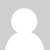### Measuring current AND voltage?

Hi,

i am trying to understand the webpage about "Arduino Math".

There is written, that the emonTX measures current and voltage from the ct.

When i look at the schematics i can only see that the ct is connected with the Arduion over a shunt and then biased

with DC. The result is voltage, which is then connected to arduino analog input.

Or is the arduino capable of measuring also current?

Olaf

### Re: Measuring current AND voltage?

Hello, Olaf and welcome.

The emonTx measures current and voltage separately.

Current is from the ct which gives 50 mA at 100 A mains current, a burden resistor of 18 Ohms converts this to a 1 V rms (approximately) voltage signal that is biassed to 1.65 V and fed into the Atmel processor analogue input.

Mains supply voltage is transformed down to 9 V with an ac-ac adapter, then divided by a potential divider (two resistors) to about the same 1 V rms and likewise biassed to 1.65 V.

The Atmel processor runs at 3.3 V so 1.65 V is half of this and so the whole wave is digitised, and from that the current, voltage, real power, apparent power and power factor can be calculated.

### Re: Measuring current AND voltage?

Hello Olaf,

The Arduino can only measure voltage at its analogue input pins.  These voltages come from sensors which are made out of hardware.  There are two different types of sensors:

1. There is a voltage sensor for measuring the voltage of the mains supply.  In the UK, we have 240Vrms at 50Hz AC.  This voltage is reduced in size by a transformer so that it is OK for connecting to the Arduino.  This type of sensor is fully described at http://openenergymonitor.org/emon/buildingblocks/measuring-voltage-with-an-acac-power-adapter

2.  There is a different kind of sensor for measuring current.  This has two main parts: first, there is the Current Transformer, or CT, which clips around the cable where AC current is flowing (this can be the Live wire, or the Neutral, it does not matter which one).  Second, there is a burden resistor (shunt?) which connects across the output coil of the CT.  This completes the circuit.  Current from the output of the CT can now flow through the burden resistor so that an AC voltage is developed across that resistor.

Both types of sensor are connected to the Arduino in the same way.  One part of each sensor is connected to a reference point which is at the mid point of the ADC's input range.  A different part of the sensor is connected the the ADC input of the Arduino.

So, although the Arduino makes two measurements of voltage, one of these is proportional to AC voltage, and one is proportional to AC current.  The best way to measure power or energy is to measure both current and voltage, and then multiply these values together (at least 2500 times every second)

I hope this helps :)### Re: Measuring current AND voltage?

Thank you very much for the explanation.

So the device can measure the current of three lines with these ct and the voltage.

But doesn't than mean, that the ct's have to be on the same phase?

Otherwise i get problems with cos phi, right?

Olaf

### Re: Measuring current AND voltage?

Olaf, the normal approach is to measure the power on just one phase,  The best way to do this is with one voltage sensor and one current sensor.  These will both be on the same phase.

If you have a 3-phase system, then you would need to measure the current on each phase separately.   To find the total power, you may need to measure the voltage on each phase separately too.  That would probably require the use of three separate processors.  Some people on the forum are working with 3-phase power, but most of us only have a single-phase supply.

I think it would be best for you to measure power on one just phase first!

Calypso

### Re: Measuring current AND voltage?

Dear all,

I'm new in this project, I have few question.

It's clear to me that we measure current with CT sensor and AC voltage via the below descriped way.

I would like to know the resolution of the Current measurement .

Where can I read abouth the calculation of the real power, apparent power and power factor ?

I mean mathematic, Electrical side and program side.

I would like to understand deeply how it's calculated.